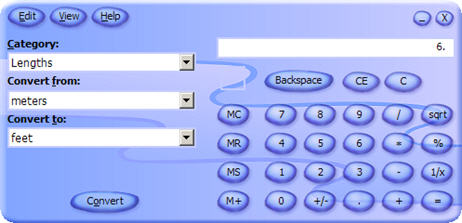Microsoft Calculator Plus: Currency, units and measurements conversionMicrosoft Calculator Plus is a free replacement of Microsoft Calculator 3.0 that ships with Windows XP. It also includes all the mathematical functions offered in Microsoft Calculator basic and some more features.

Microsoft Calculator Plus lets you convert between different measurement units for area, temperature, volume and currency. Microsoft Calculator Plus contains legacy Euro currencies with predefined rates. Latest exchange rates are downloaded from the European Central Bank.

All mathematical operations in Microsoft Calculator Plus are accurate to at least 32 digits. For example, 1/3 is stored as 1/3, rather than .333.

Microsoft Calculator Plus also performs basic arithmetic, such as addition and subtraction, as well as functions found on a scientific calculator, such as logarithms and factorials.